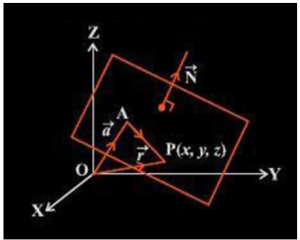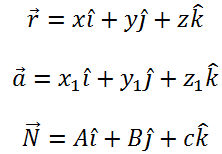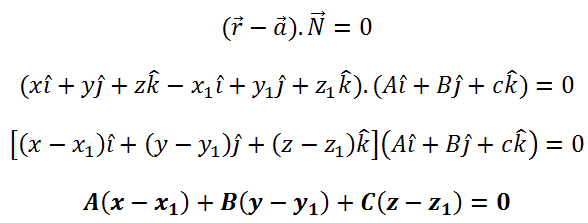# Equation of A Plane

A Vector is a physical quantity that with it’s magnitude also has a direction attached to it. With reference to a origin the position vector basically denotes the location or position (in a 3D Cartesian system) of a point. The Cartesian equation of a plan in 3 Dimensional space and vectors are explored in this post.

## Equation of a plane

There are infinite planes that lie perpendicular to a specific vector. But only one unique plane exists to a specific point which remains perpendicular to the point while going through it.

Let us consider a plane passing through a given point A having position vector   $\vec{a}$ and perpendicular to the vector  $\vec{N}$ . Let us consider a point P(x, y, z) lying on this plane and its position vector is given by  $\vec{r}$ as shown in the figure given below.Position vector simply denotes the position or location of a point in the three dimensional Cartesian system with respect to a reference origin.

For point P to lie on the given plane it must satisfy the following condition:

$\vec{AP}$  is perpendicular to $\vec{N}$  , i.e. $\vec{AP}$.$\vec{N}$ =0

From the figure given above it can be seen that,

$\vec{AP}$ = ( $\vec{r}$ – $\vec{a}$)

Substituting this value in  $\vec{AP}$$\vec{N}$ =0, we have ($\vec{r}$ – $\vec{a}$). $\vec{N}$ =0

This equation represents the vector equation of a plane.

We will assume that P, Q and R points are regarded as x1, y1, z1 and x2, y2, z2 in respectively to change the equation into Cartesian system. A, B and C will be the assumed direction ratios. Thus,Substituting these values in the vector equation of a plane, we haveThis gives us the Cartesian equation of a plane. To learn more about equation of a plane in three dimensions and three dimensional geometry download Byju’s- The Learning App.